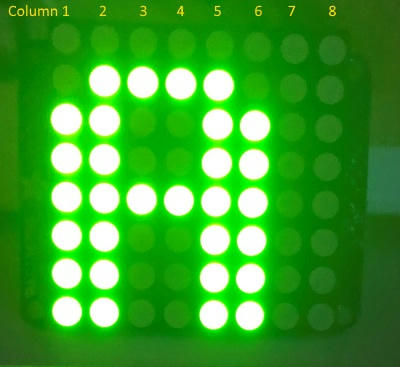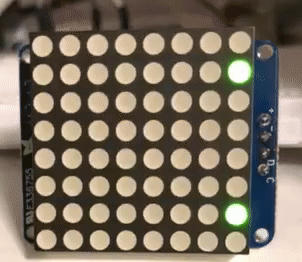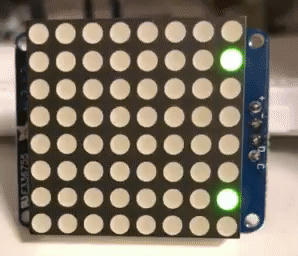# 8x8 LED Matrix Offset by 1

Hello Everyone,
I am working with Xojo on a Raspberry Pi 3 B+ to power an 8x8 LED Matrix with a I2C HT16K33 backpack (https://www.adafruit.com/product/871). The good news is that I can get all of the pins to light up, the weird part is that all of the columns are offset by 1. How do I have the centered code work with a centered letter on the 8x8 matrix?

When I light up the letter A in my code, the code should have the letter with a space on either side of the letter that is not lit by LEDs:
&b00000000
&b00111100
&b01100110
&b01100110
&b01111110
&b01100110
&b01100110
&b01100110

Col12345678 (columns)
The actual result on the LED is:

00000000
01111000
11001100
11001100
11111100
11001100
11001100
11001100

Col23456781 (Columns)

The first column of the code is placed in column 8 on the LED.

I created a zipped file where the letter ‘A’ is centered in code and is offset on the 8x8 matrix, and then created an ‘A’’ (A-prime) example where I shifted the bits one to the right in code, where it appears to be centered but the 8th column doesnt show becuase it contains column 1 LED data. Here is the zipped Xojo file:
]Example8x8.zip

This is a picture of the LED that is centered in code and is offset on the 8x8 matrix.I might have misread the post Eugene, is this a demo or are you after a hardware or a software fix for the offset or something else?

If its a software fix, can’t you just shift all the bits across?

```Public Function RotateRight(value as UInt8) as UInt8 If BitAnd(value, 1) = 1 Then Return ShiftRight(value, 1) + 128 Else Return ShiftRight(value, 1) End If End Function```

I am likely coding something incorrectly with the hardware as one-right-bit-shift will work to centre the letter A and will work with most static letters. I ran a program written in C and it seems to be working correctly without an offset.

The problem happens when scrolling text. The LED Column 8 doesn’t light up when two right-bit-shifts occur. I could convert column 8 data to column 1 data.

I’ll need to spend more time with the C-code and 8x8 matrix datasheet to create the correct driver for the LED.

Thanks for your suggestion and taking the time to respond.You say that the output is:

[quote=393736:@Eugene Dakin]00000000
01111000
11001100
11001100
11111100
11001100
11001100
11001100[/quote]
But the picture of your LED shows column 1 on the left. Are bits vs columns really flipped?

Another possibility, could it be that youre supposed to pass a 9-bit number? So that columns 1-8 are actually bits 1-8 (instead of 0-7)

Good questions Julian and Greg,

Its almost like the starting bit needs to be at -1 instead of 0. Here is a video of an animated 8x8 LED that shifts bits to the left and shows the letter X.When I use Julians RotateRight function, then the letters starting position is correct and column 8 shows offset data.Here is the code with Julians RotateRight function:

[code]Sub Action() Handles Action
Dim Data as Integer
Data = GPIO.I2CWrite(FileHandle, &h21) //oscillate
Data = GPIO.I2CWrite(FileHandle, &hEF) //Full brightness
Data = GPIO.I2CWrite(FileHandle, &h81) //Display on

//Create the Letter A
Dim Row1 as UInt8 = RotateRight(&b10000001)
Dim Row2 as UInt8 = RotateRight(&b01000010)
Dim Row3 as UInt8 = RotateRight(&b00100100)
Dim Row4 as UInt8 = RotateRight(&b00011000)
Dim Row5 as UInt8 = RotateRight(&b00011000)
Dim Row6 as UInt8 = RotateRight(&b00100100)
Dim Row7 as UInt8 = RotateRight(&b01000010)
Dim Row8 as UInt8 = RotateRight(&b10000001)

//timeto take video
GPIO.Delay(3000)

//Show the letter A on the 8x8 LED Matrix
Dim i as integer
For i = 0 to 7
GPIO.Delay(1000)
Data = GPIO.I2CWriteReg8(FileHandle, &h0, Row8)
GPIO.Delay(10)
Data = GPIO.I2CWriteReg8(FileHandle, &h2, Row7)
GPIO.Delay(10)
Data = GPIO.I2CWriteReg8(FileHandle, &h4, Row6)
GPIO.Delay(10)
Data = GPIO.I2CWriteReg8(FileHandle, &h6, Row5)
GPIO.Delay(10)
Data = GPIO.I2CWriteReg8(FileHandle, &h8, Row4)
GPIO.Delay(10)
Data = GPIO.I2CWriteReg8(FileHandle, &hA, Row3)
GPIO.Delay(10)
Data = GPIO.I2CWriteReg8(FileHandle, &hC, Row2)
GPIO.Delay(10)
Data = GPIO.I2CWriteReg8(FileHandle, &hE, Row1)

``````Row1 = ShiftLeft(Row1,1)
Row2 = ShiftLeft(Row2,1)
Row3 = ShiftLeft(Row3,1)
Row4 = ShiftLeft(Row4,1)
Row5 = ShiftLeft(Row5,1)
Row6 = ShiftLeft(Row6,1)
Row7 = ShiftLeft(Row7,1)
Row8 = ShiftLeft(Row8,1)
``````

Next i
End Sub
[/code]

A good suggestion. I tried the above example with 9-bits instead, and had similar results with column 8 causing grief.

LOL, this is a head-scratcher. I may just need to code it differently to compensate for column 8. It would be kind-of a conversion for the program to treat column 8 like a column -1.

Try it this way:

[code]Dim Data As Integer
Data = GPIO.I2CWrite(FileHandle, &h21) //oscillate
Data = GPIO.I2CWrite(FileHandle, &hEF) //Full brightness
Data = GPIO.I2CWrite(FileHandle, &h81) //Display on

//Create the Letter A
Dim Row1 As UInt8 = &b10000001
Dim Row2 As UInt8 = &b01000010
Dim Row3 As UInt8 = &b00100100
Dim Row4 As UInt8 = &b00011000
Dim Row5 As UInt8 = &b00011000
Dim Row6 As UInt8 = &b00100100
Dim Row7 As UInt8 = &b01000010
Dim Row8 As UInt8 = &b10000001

//timeto take video
GPIO.Delay(3000)

//Show the letter A on the 8x8 LED Matrix
Dim i As Integer
For i = 0 To 7
GPIO.Delay(1000)
Data = GPIO.I2CWriteReg8(FileHandle, &h0, RotateRight(Row8))
GPIO.Delay(10)
Data = GPIO.I2CWriteReg8(FileHandle, &h2, RotateRight(Row7))
GPIO.Delay(10)
Data = GPIO.I2CWriteReg8(FileHandle, &h4, RotateRight(Row6))
GPIO.Delay(10)
Data = GPIO.I2CWriteReg8(FileHandle, &h6, RotateRight(Row5))
GPIO.Delay(10)
Data = GPIO.I2CWriteReg8(FileHandle, &h8, RotateRight(Row4))
GPIO.Delay(10)
Data = GPIO.I2CWriteReg8(FileHandle, &hA, RotateRight(Row3))
GPIO.Delay(10)
Data = GPIO.I2CWriteReg8(FileHandle, &hC, RotateRight(Row2))
GPIO.Delay(10)
Data = GPIO.I2CWriteReg8(FileHandle, &hE, RotateRight(Row1))

Row1 = ShiftLeft(Row1, 1)
Row2 = ShiftLeft(Row2, 1)
Row3 = ShiftLeft(Row3, 1)
Row4 = ShiftLeft(Row4, 1)
Row5 = ShiftLeft(Row5, 1)
Row6 = ShiftLeft(Row6, 1)
Row7 = ShiftLeft(Row7, 1)
Row8 = ShiftLeft(Row8, 1)
Next i[/code]

Blessed are you who are having fun !
I still could not buy my raspberry PI, but I think I will do it in the future.
I would like to build a cash register for use with my erp software.
however I will keep this post aside because I will need it

Julian, you are great! It works.

My next question is why does it work? Is it possible that the UInt8 values were defaulting to another datatype, like an Int8 or a UInt16? I am interested in your thoughts.

Thank you!

No probs. I don’t know why the offset is needed without looking into the datasheet etc. but the reason why the rotation was messing up was because you were using the rotated values in your loop rather than rotating them just before and only for output.Face-palm. Of course. Thank you Julian.